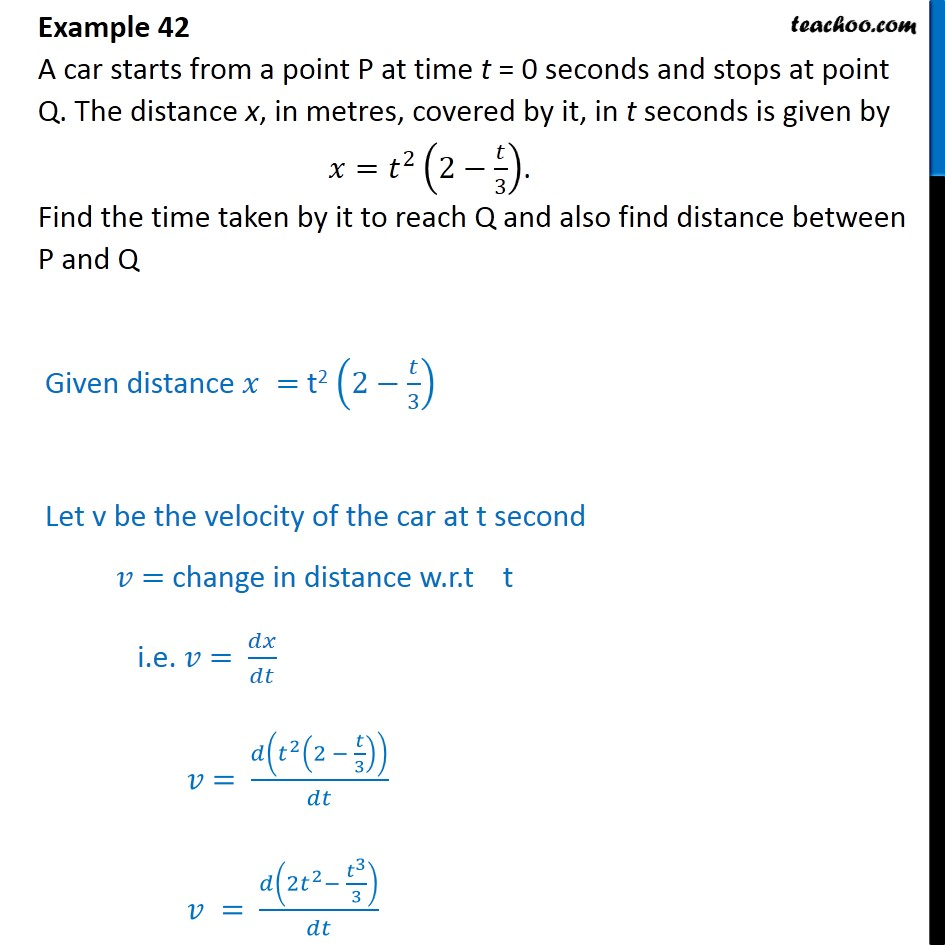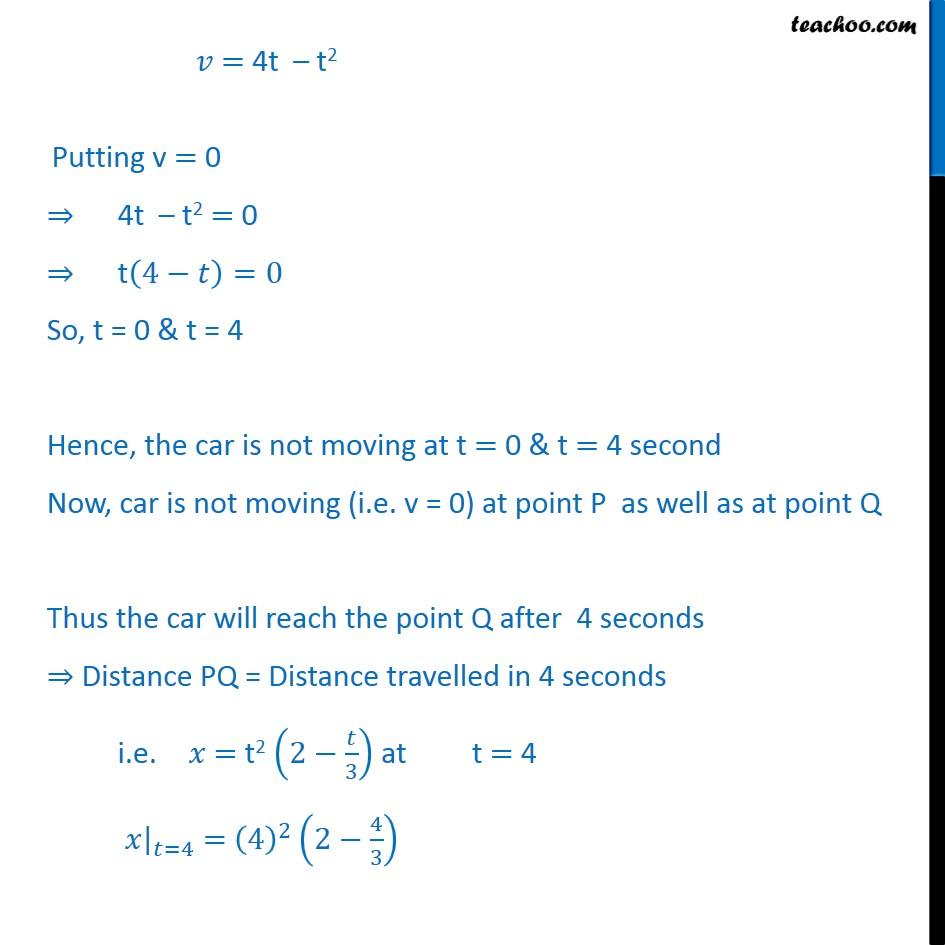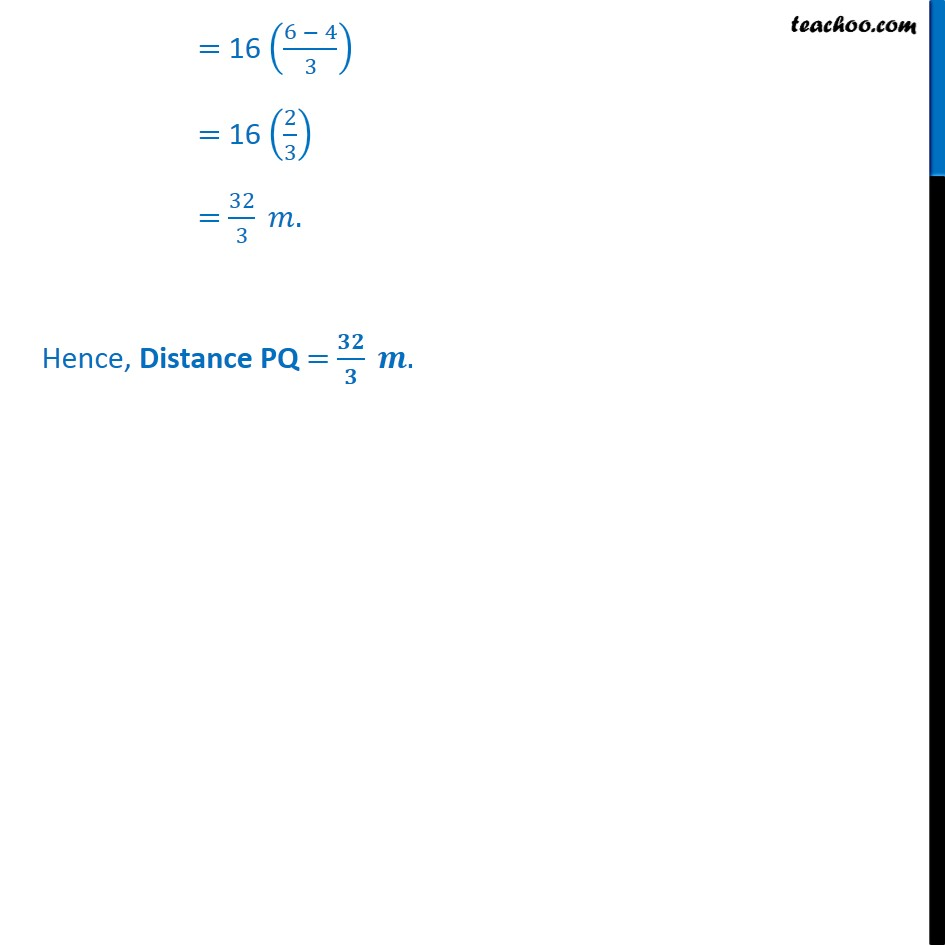1. Chapter 6 Class 12 Application of Derivatives
2. Serial order wise
3. Examples

Transcript

Example 42 A car starts from a point P at time t = 0 seconds and stops at point Q. The distance x, in metres, covered by it, in t seconds is given by = 2 2 3 . Find the time taken by it to reach Q and also find distance between P and Q Given distance = t2 2 3 Let v be the velocity of the car at t second = change in distance w.r.t t i.e. = = 2 2 3 = 2 2 3 3 = 4t t2 Putting v = 0 4t t2 = 0 t 4 =0 So, t = 0 & t = 4 Hence, the car is not moving at t = 0 & t = 4 second Now, car is not moving (i.e. v = 0) at point P as well as at point Q Thus the car will reach the point Q after 4 seconds Distance PQ = Distance travelled in 4 seconds i.e. = t2 2 3 at t = 4 =4 = 4 2 2 4 3 = 16 6 4 3 = 16 2 3 = 32 3 . Hence, Distance PQ = .

Examples